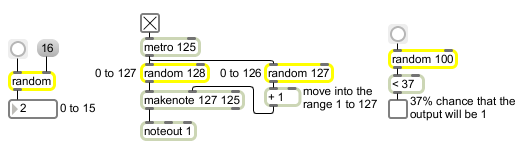random

Generate a random number

Description

random acts as a random number generator which will output random numbers within the range between 0 and 1 less than the argument specified.

Arguments

Name Type Opt Description
initial-maximum-limit int opt Sets an initial limit to the random output. The output will always be between 0 and one less than this maximum limit. If there is no argument, the limit is initially set to 1, which causes random to output 0 whenever it receives a bang.
seed-value int opt A second argument is used to set a "seed" value for the random generator. If no argument is specified, the time value will be used to initialize the seed.

Messages

 bang In left inlet: Sends out a randomly generated number between 0 and one less than its maximum limit. seed seed-value [int] In left inlet: The word seed, followed by a number, provides a "seed" value for the random generator, which causes a specific (reproducible) sequence of pseudo-random numbers to occur. The number 0 uses the time elapsed since system startup (an unpredictable value) as the seed, ensuring an unpredictable sequence of numbers. This unpredictable seed is used by default when the random object is created.

Output

int: When a bang is received in the left inlet, random generates a random number between 0 and one less than its maximum limit.

ExamplesGenerate random events or make decisions based on probability